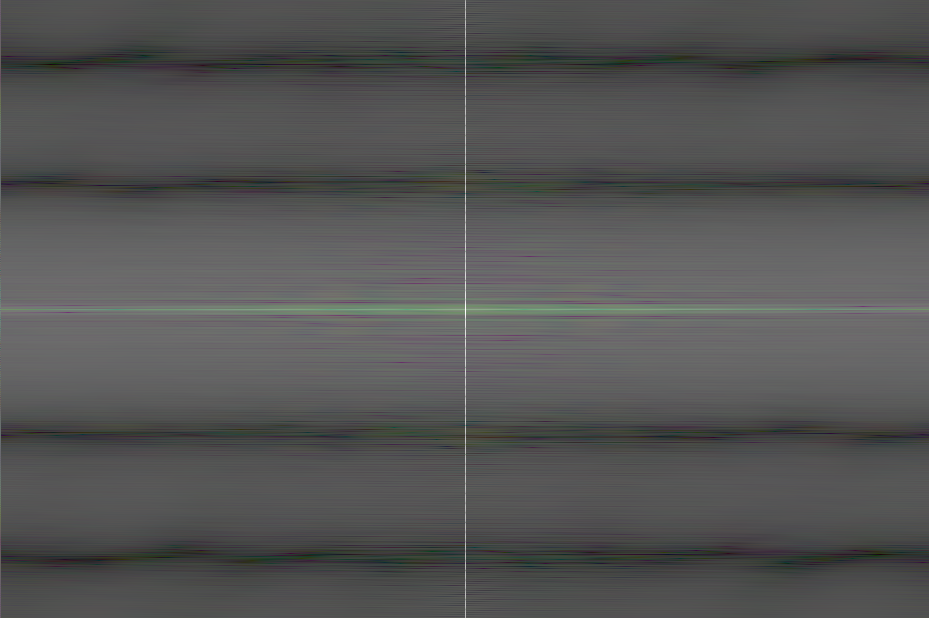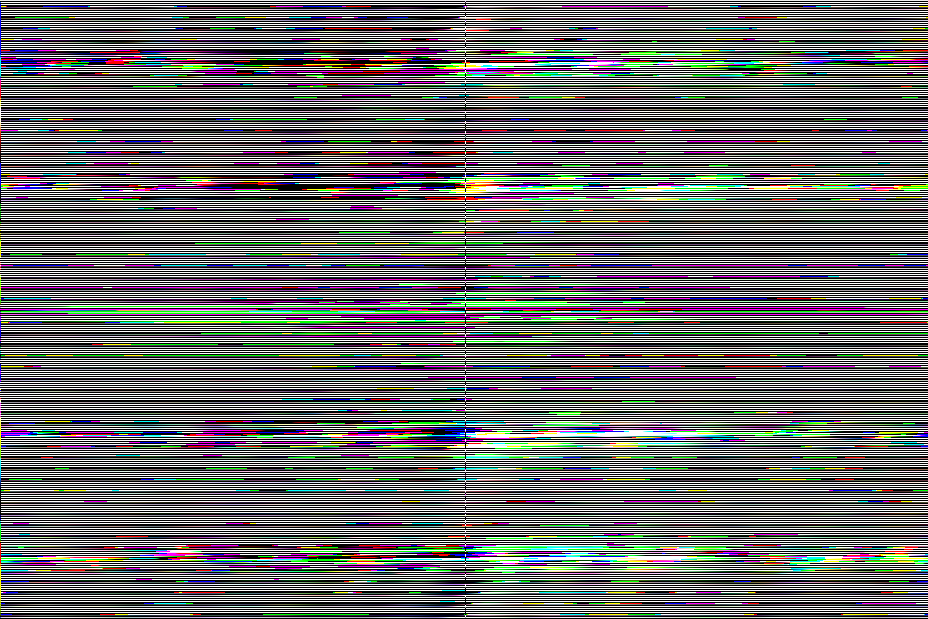Tuesday, April 21, 2015

2D FFT: Effects of Location & Thickness of Horizontal Lines on 2D FFT Magnitude & Phase: Part 04

Problem

This post continues our series of posts on a programmatic investigation of the effects of the location and thickness of horizontal, vertical, and diagonal lines on the magnitude and phase components of 2D FFT. In this post, we will continue our investigation of horizontal lines by modifying their thickness and position in the image. The previous post is here.

Thickened Horizontal Line Shifted Upward

Figure 1 shows a thickened horizontal line shifted upward in the image.Figure 1. Thickened horizontal line shifted upward (hor_line_01e.jpg)

figure;
imshow(img_hor_line_01e);
title('HOR LINE 01e');

Here is how we compute the 2D FFT of the image. The image is converted into a 2D double matrix. The fftshift command positions the 0,0 origin to the center of the image. We compute the absolute magnitude of each value in the 2D matrix and take the log of each value. 1 is added to each value before taking the log, because log(0) is not defined.

%% ff2 magnitude
fftHorLine01e = fft2(double(img_hor_line_01e));
fftHorLine01e = fftshift(fftHorLine01e);
fftHorLine01e = abs(fftHorLine01e);
fftHorLine01e = log(fftHorLine01e+1);
fftHorLine01e = mat2gray(fftHorLine01e);

The magnitude image is shown in Figure 2. As can be seen in Figure 2, the FFT magnitude shows a line orthogonal to the line in Figure 1.

figure;
imshow(fftHorLine01e), colormap(gray);
title('HOR LINE 01e FFT2 Magnitude');Figure 2. 2D FFT magnitude of Figure 1

We compute the phase component and display it. The image is shown in Figure 3.

%% fft2 phase
fftHorLine01e_PHASE = fft2(double(img_hor_line_01e));
fftHorLine01e_PHASE = fftshift(fftHorLine01e_PHASE);
fftHorLine01e_PHASE = angle(fftHorLine01e_PHASE);

figure;
imshow(fftHorLine01e_PHASE, [-pi, pi]), colormap(gray);
title('HOR LINE 01e FFT2 Phase');Figure 3. 2D FFT phase of Figure 1

The complete  MATLAB source code is in this post. The next post begins our investigation of vertical lines.